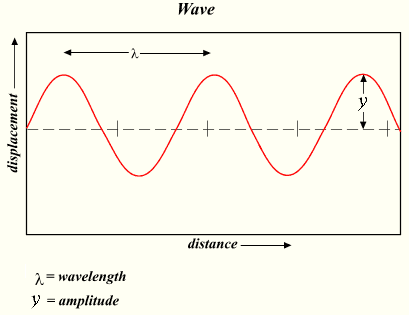# Traveling Wave Relationship

The relationship of the wave frequency, wavelength and propagation velocity.If you throw a rock into the water, this will create a wave. The wave would travel outward from the point where the rock hit the water. If propagation velocity is a constant, traveled distance will be velocity multiplied by time. Wavelength is the distance traveled by wave for the time equals to wave period.
Thus, to find out wavelength, we need to multiply propagation velocity by period:

Using the definition of frequency as

,

we can get standard wave relationship:

The calculator below computes unknown parameters using two known parameters.#### Traveling Wave Relationship

Digits after the decimal point: 3
Velocity, m/s

Wavelength, m

Period, s

Frequency, Hz

URL copied to clipboard
PLANETCALC, Traveling Wave Relationship